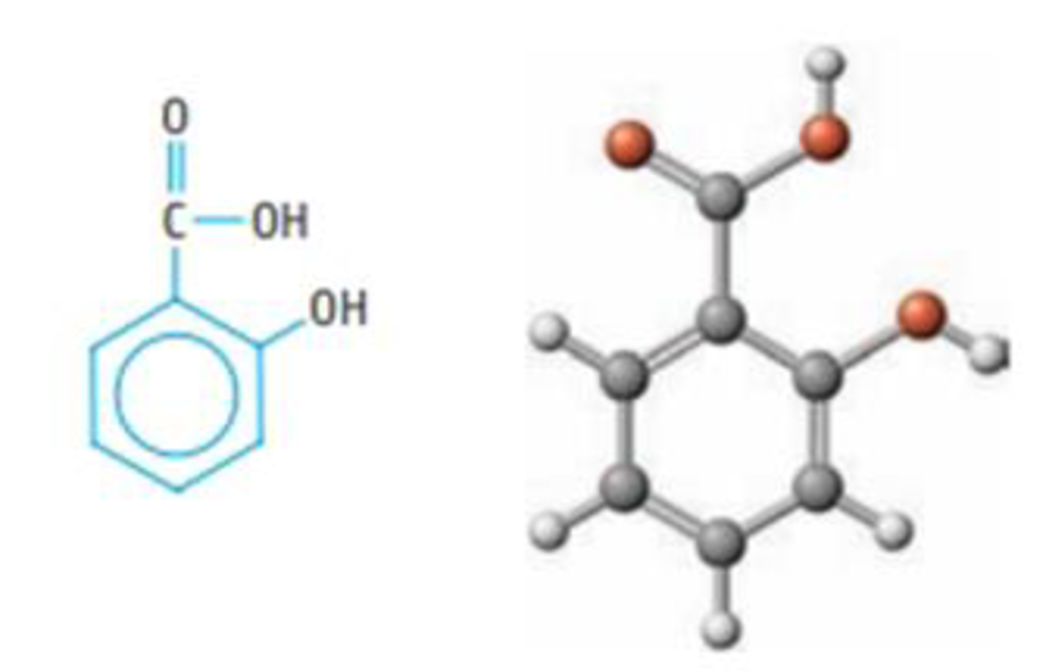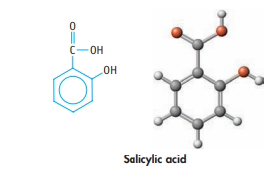# The chemical name for aspirin is acetylsalicylic acid. It is believed that the analgesic and other desirable properties of aspirin are due not to the aspirin itself but rather to the simpler compound salicylic add, C 6 H 4 (OH)CO 2 H, which results from the breakdown of asp inn in the stomach. Salicylic acid (a) Give approximate values for the following bond angles in the acid: (i) C—C—C in the ring, (ii) O—C=O; (iii) either of the C—O—H angles: and (iv) C—C—H. (b) What is the hybridization of the C atoms of the ring? Of the C atom in the —CO 2 H group? (c) Experiment shows that 1.00 g of the acid will dissolve in 460 ml of water. If the pH of this solution is 2.4, what is K a for the acid? (d) If you have salicylic acid in your stomach and if the pH of gastric juice is 2.0, calculate the percentage of salicylic add that will be present in the stomach in the form of the salicylate ion, C 6 H 4 (OH)CO 2 − . (e) Assume you have 25.0 mL of a 0.014 M solution of salicylic acid and titrate it with 0.010 M NaOH. What is the pH at the halfway point of the titration? What is the pH at the equivalence point?### Chemistry & Chemical Reactivity

9th Edition
John C. Kotz + 3 others
Publisher: Cengage Learning
ISBN: 9781133949640

#### Solutions

Chapter
Section### Chemistry & Chemical Reactivity

9th Edition
John C. Kotz + 3 others
Publisher: Cengage Learning
ISBN: 9781133949640
Chapter 17, Problem 119SCQ
Textbook Problem
5 views

## The chemical name for aspirin is acetylsalicylic acid. It is believed that the analgesic and other desirable properties of aspirin are due not to the aspirin itself but rather to the simpler compound salicylic add, C6H4(OH)CO2H, which results from the breakdown of asp inn in the stomach.Salicylic acid (a) Give approximate values for the following bond angles in the acid: (i) C—C—C in the ring, (ii) O—C=O; (iii) either of the C—O—H angles: and (iv) C—C—H. (b) What is the hybridization of the C atoms of the ring? Of the C atom in the —CO2H group? (c) Experiment shows that 1.00 g of the acid will dissolve in 460 ml of water. If the pH of this solution is 2.4, what is Ka for the acid? (d) If you have salicylic acid in your stomach and if the pH of gastric juice is 2.0, calculate the percentage of salicylic add that will be present in the stomach in the form of the salicylate ion, C6H4(OH)CO2−. (e) Assume you have 25.0 mL of a 0.014 M solution of salicylic acid and titrate it with 0.010 M NaOH. What is the pH at the halfway point of the titration? What is the pH at the equivalence point?

a)

Interpretation Introduction

Interpretation:

The following has to be determined.

1. (a) Write the approximate values for the following bond angles in the acid.

(i)   C - C - C in ring(ii)  O - C = O(iii) C - C - H angles(iv)  C - C - H

Concept introduction:

The chemical name for aspirin is acetylsalicylic acid. It is believed that analgesic and other desirable properties of aspirin are due not to the aspirin itself rather to the simpler compound salicylic acid, C6H4(OH)CO2H, which results from the breakdown of aspirin in the stomach.

The structure is as follows.### Explanation of Solution

1. (i) Each carbon atom is attached to the two carbon atoms either hydrogen atom or one or one of the two substituent. And each carbon of the ring has electron – pair geometry of trigonal planar. A trigonal planar atom has bond angles of 1200.

Therefore, C - C - C bond angle is 1200

2. (ii) The carbon attached to the two oxygen atoms ha electron pair geometry of trigonal planar because it is attached to two oxygen atoms and one carbon atom. A trigonal planar atom ha bond angles of 1200.

Therefore, O - C = O bond angle is 1200.

3. (iii) The oxygen attached to the carbon and the hydrogen atoms has electron –pair geometry of tetrahedral because it is attached to two atoms and has two lone pairs

b)

Interpretation Introduction

Interpretation:

The hybridization of the C atoms of the ring; and of the C atom in the - CO2H group following has to be determined

Concept introduction:

The chemical name for aspirin is acetylsalicylic acid. It is believed that analgesic and other desirable properties of aspirin are due not to the aspirin itself rather to the simpler compound salicylic acid, C6H4(OH)CO2H, which results from the breakdown of aspirin in the stomach.

The structure is as follows.c)

Interpretation Introduction

Interpretation:

Ka for the acid. If the pH of the solution is 2.4 has to be determined.

Concept introduction:

The chemical name for aspirin is acetylsalicylic acid. It is believed that analgesic and other desirable properties of aspirin are due not to the aspirin itself rather to the simpler compound salicylic acid, C6H4(OH)CO2H, which results from the breakdown of aspirin in the stomach.

The structure is as follows.d)

Interpretation Introduction

Interpretation:

The percentage of salicylic acid if the pH of the of gastric juice is 2.0. And the form of salicylate ion, C6H4(OH)CO2- has to be calculated.

Concept introduction:

The chemical name for aspirin is acetylsalicylic acid. It is believed that analgesic and other desirable properties of aspirin are due not to the aspirin itself rather to the simpler compound salicylic acid, C6H4(OH)CO2H, which results from the breakdown of aspirin in the stomach.

The structure is as follows.e)

Interpretation Introduction

Interpretation:

The pH at the halfway point of the titration and pH at the equivalence point has to be determined

Concept introduction:

The chemical name for aspirin is acetylsalicylic acid. It is believed that analgesic and other desirable properties of aspirin are due not to the aspirin itself rather to the simpler compound salicylic acid, C6H4(OH)CO2H, which results from the breakdown of aspirin in the stomach.

The structure is as follows.### Still sussing out bartleby?

Check out a sample textbook solution.

See a sample solution

#### The Solution to Your Study Problems

Bartleby provides explanations to thousands of textbook problems written by our experts, many with advanced degrees!

Get Started

Find more solutions based on key concepts
For healthy adults, the DRI recommended intake for protein has been set at 0.3 grams per kilogram of body weigh...

Nutrition: Concepts and Controversies - Standalone book (MindTap Course List)

How can a star of as much as 8 solar masses form a white dwarf when it dies?

Horizons: Exploring the Universe (MindTap Course List)

Three wires are made of copper having circular cross sections. Wire 1 has a length L and radius r. Wire 2 has a...

Physics for Scientists and Engineers, Technology Update (No access codes included)

What is compensation depth?

Oceanography: An Invitation To Marine Science, Loose-leaf Versin